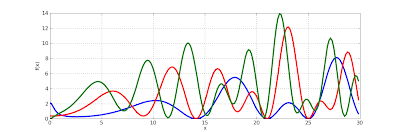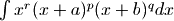# Hurwitz zeta function, Dirichlet L-series, Appell F1

July 14, 2009

I’ve added three more functions to mpmath since the last blog update: the Hurwitz zeta function, Dirichlet L-series and the Appell hypergeometric function.

#### Hurwitz zeta function

The Hurwitz zeta function is available with the syntax hurwitz(s, a=1, derivative=0). It’s a separate function from zeta for various reasons (one being to make the plain zeta function as simple as possible). With the optional third argument, it not only computes values, but arbitrarily high derivatives with respect to s (more on which later).

It’s quite fast at high precision; e.g. the following evaluation takes just 0.25 seconds (0.6 seconds from cold cache) on my laptop:

>>> mp.dps = 1000>>> hurwitz(3, (1,3))        # (1,3) specifies the exact parameter 1/327.56106119970080377622787797740750928454209531301488108286446031855695818017193319714478144925225572428339288398963479601630790061026511179978568818123994067840573189334947537240910125121134668254531419227282374538466526604902207819804397999746337871597509019667252833751410875882452970087673701166818534638337151352084773537656137422271798879545153523879812143981699853880764694094326889123555060920043334937078540793636560719603896550074378037796559947176109732627192261674381230503180321801486524844073873393780084553765789276561564723000726671702263215399293512078780185479463617569113965545079189333448241385996978516660755944879572783482345047875282167516302446341564720560895973910007102633691282693068326115586706265136825191025873785920661492516925137079914816949085911457084632783280741306988974583447464692728282674849897565952599172908008487680740297664177868157329190791734974760032062614100444842998745085439117586813879301569134308141720962503488644344900903488585429308918210445984048

With s = π instead, it takes 3.5 seconds. For comparison, Mathematica 6 took 0.5 and 8.3 seconds respectively (on an unloaded remote system which is faster than my laptop, but I’m not going to guess by how much).

The function is a bit slow at low precision, relatively speaking, but fast enough (in the 1-10 millisecond range) for plotting and such. Of course, it works for complex s, and in particular on the critical line 1/2+it. Below, I’ve plotted |ζ(1/2+it,1)|2, |ζ(1/2+it,1/2)|2, |ζ(1/2+it,1/3)|2; the first image shows 0 ≤ t < 30 and the second is with 1000 ≤ t < 1030.To show some more complex values, here are plots of ζ(s, 1), ζ(s, 1/3) and ζ(s, 24/25) for 100 ≤ Im(s) ≤ 110. I picked the range [-2, 3] for the real part to show that the reflection formula for Re(s) < 0 works:In fact, the Hurwitz zeta implementation works for complex values of both s and a. Here is a plot of hurwitz(3+4j, a) with respect to a:The evaluation can be slow and/or inaccurate for nonrational values of a, though (in nonconvergent cases where the functional equation isn’t used).

#### Zeta function performance

Right now the implementations of the Riemann zeta function and the Hurwitz zeta function in mpmath are entirely separate. In fact, they even use entirely different algorithms (hurwitz uses Euler-Maclaurin summation, while zeta uses the Borwein approximation). This is useful for verifying correctness of either function.

Regarding performance, it appears that the Borwein approximation is slightly faster for small |Im(s)| while Euler-Maclaurin is massively faster for large |s|.

The following benchmark might give an idea:

>>> from mpmath import *>>> mp.dps = 15; mp.pretty = True>>> timing(zeta, 0.5+10j); timing(hurwitz, 0.5+10j)0.00273861622077666270.0082901877193889192>>> timing(zeta, 0.5+100j); timing(hurwitz, 0.5+100j)0.00753193492469010890.041037198861866478>>> timing(zeta, 0.5+1000j); timing(hurwitz, 0.5+1000j)0.134684994880634790.18062463246027072>>> timing(zeta, 0.5+10000j); timing(hurwitz, 0.5+10000j)7.29775935209642411.0488757649366072>>> timing(zeta, 0.5+10000j); timing(hurwitz, 0.5+10000j)0.843066367373509711.0601629536714867

As the last evaluation shows, the Borwein algorithm is still not doing too poorly at 0.5+10000j from a warm cache, but at this point the cache is 50 MB large. At 0.5+100000j my laptop ran out of RAM and Firefox crashed (fortunately Blogger autosaves!) so it clearly doesn’t scale. The Euler-Maclaurin algorithm caches a lot of Bernoulli numbers, but the memory consumption of this appears to be negligible. With hurwitz (and the function value, for those interested),

>>> timing(hurwitz, 0.5+100000j)8.0361576680835149>>> hurwitz(0.5+100000j)(1.07303201485775 + 5.7808485443635j)

and the memory usage of the Python interpreter process only climbs to 12 MB.

Just by optimizing the pure Python code, I think the Hurwitz zeta function (at low precision) could be improved by about a factor 2 generally and perhaps by a factor 3-4 on the critical line (where square roots can be used instead of logarithms — zeta uses this optimization but hurwitz doesn’t yet); with Cython, perhaps by as much as a factor 10.

The real way to performance is not to use arbitrary-precision arithmetic, though. Euler-Maclaurin summation for zeta functions is remarkably stable in fixed-precision arithmetic, so there is no problem using doubles for most applications. As I wrote a while back on sage-devel, a preliminary version of my Hurwitz zeta code for Python complex was 5x faster than Sage’s CDF zeta (in a single test, mind you). If there is interest, I could add such a version, perhaps writing it in Cython for Sage (for even greater speed).

#### Derivatives

The Hurwitz zeta function can be differentiated easily like so:

>>> mp.dps = 25>>> hurwitz(2+3j, 1.25, 3)(-0.01266157985314340398338543 - 0.06298953579777517606036907j)>>> diff(lambda s: hurwitz(s, 1.25), 2+3j, 3)(-0.01266157985314340398338543 - 0.06298953579777517606036907j)

For simple applications one can just as well use the numerical diff function as above with reasonable performance, but this isn’t really feasible at high precision and/or high orders (numerically computing an nth derivative at precision p requires n+1 function evaluations, each at precision (n+1)×p).

The specialized code in hurwitz requires no extra precision (although it still needs to perform extra work) and therefore scales up to very high precision and high orders. Here for example is a derivative of order 100 (taking 0.5 seconds) and one of order 200 (taking 4 seconds):

>>> hurwitz(2+3j, 1.25, 100)(2.604086240825183469410664e+107 - 1.388710675207202633247271e+107j)>>> hurwitz(2+3j, 1.25, 200)(2.404124816484789309285653e+274 + 6.633220818921777955999455e+273j)

It should be possible to calculate a sequence of derivatives much more quickly than with separate calls, although this isn’t implemented yet. One use for this is to produce high-degree Taylor series. This could potentially be used in a future version of mpmath, for example to provide very fast moderate-precision (up to 50 digits, say) function for multi-evaluation of the Riemann zeta function on the real line or not too far away from the real line. This in turn would speed up other functions, such as the prime zeta function and perhaps polylogarithms in some cases, which are computed using series over many Riemann zeta values.

#### Dirichlet L-series

With the Hurwitz zeta function in place, it was a piece of cake to also supply an evaluation routine for arbitrary Dirichlet L-series.

The way it works it that you provide the character explicitly as a list (so it also works for other periodic sequences than Dirichlet characters), and it evaluates it as a sum of Hurwitz zeta values.

For example (copypasting from the docstring), the following defines and verifies some values of the Dirichlet beta function, which is defined by a Dirichlet character modulo 4:

>>> B = lambda s, d=0: dirichlet(s, [0, 1, 0, -1], d)>>> B(0); 1./20.50.5>>> B(1); pi/40.78539816339744830961566090.7853981633974483096156609>>> B(2); +catalan0.91596559417721901505460350.9159655941772190150546035>>> B(2,1); diff(B, 2)0.081580736116592795102912170.08158073611659279510291217>>> B(-1,1); 2*catalan/pi0.58312180806163756027676890.5831218080616375602767689>>> B(0,1); log(gamma(0.25)**2/(2*pi*sqrt(2)))0.39159439270683677647194530.3915943927068367764719454>>> B(1,1); 0.25*pi*(euler+2*ln2+3*ln(pi)-4*ln(gamma(0.25)))0.19290131679691242936318980.1929013167969124293631898

#### Appell hypergeometric function

The function appellf1 computes the Appell F1 function which is a hypergeometric series in two variables. I made the implementation reasonably fast by rewriting it as a single series in hyp2f1 (with the side effect of supporting the analytic continuation with that for 2F1).

A useful feature of the Appell F1 function is that it provides a closed form for some integrals, including those of the formwith arbitrary parameter values, for arbitrary endpoints (and that’s a quite general integral indeed). Comparing with numerical quadrature:

>>> def integral(a,b,p,q,r,x1,x2):...     a,b,p,q,r,x1,x2 = map(mpmathify, [a,b,p,q,r,x1,x2])...     f = lambda x: x**r * (x+a)**p * (x+b)**q...     def F(x):...         v = x**(r+1)/(r+1) * (a+x)**p * (b+x)**q...         v *= (1+x/a)**(-p)...         v *= (1+x/b)**(-q)...         v *= appellf1(r+1,-p,-q,2+r,-x/a,-x/b)...         return v...     print "Num. quad:", quad(f, [x1,x2])...     print "Appell F1:", F(x2)-F(x1)...>>> integral('1/5','4/3','-2','3','1/2',0,1)Num. quad: 9.073335358785776206576981Appell F1: 9.073335358785776206576981>>> integral('3/2','4/3','-2','3','1/2',0,1)Num. quad: 1.092829171999626454344678Appell F1: 1.092829171999626454344678>>> integral('3/2','4/3','-2','3','1/2',12,25)Num. quad: 1106.323225040235116498927Appell F1: 1106.323225040235116498927

Also incomplete elliptic integrals are covered, so you can for example define one like this:

>>> def E(z, m):...     if (pi/2).ae(z):...         return ellipe(m)...     return 2*round(re(z)/pi)*ellipe(m) + mpf(-1)**round(re(z)/pi)*\...         sin(z)*appellf1(0.5,0.5,-0.5,1.5,sin(z)**2,m*sin(z)**2)...>>> z, m = 1, 0.5>>> E(z,m); quad(lambda t: sqrt(1-m*sin(t)**2), [0,pi/4,3*pi/4,z])0.92732988362444006696590420.9273298836244400669659042>>> z, m = 3, 2>>> E(z,m); quad(lambda t: sqrt(1-m*sin(t)**2), [0,pi/4,3*pi/4,z])(1.057495752337234229715836 + 1.198140234735592207439922j)(1.057495752337234229715836 + 1.198140234735592207439922j)

The Appell series isn’t really faster than numerical quadrature, but it’s possibly more robust (the singular points need to be given to quad as above to obtain any accuracy, for example). Mpmath doesn’t yet have incomplete elliptic integrals; the version above could be a start, although I’ll probably want to try a more canonical approach.